# Oberseminar Analysis Summer 2022

## Previous weeks:

• Thursday, May 12 at 14:15 in SemR 0.011
Arianna Giunti (Imperial College London)
Quantitative homogenization for interacting particle systems

We are interested in the bulk diffusion matrix $\bar a$ associated to a class of interacting particle systems in $\mathbb R^{d}$. The models that we consider are reversible with respect to the Poisson measures and are of non-gradient type.

The matrix $\bar a$ may be thought as the effective diffusion of a cloud of interacting particles. Relying on the recent progress in quantitative stochastic homogenization for elliptic PDEs, we prove that finite-volume approximations of $\bar a$ converge at an algebraic rate. Furthermore, we show that $\bar a$ is smooth in the density of particles and we characterise its derivatives.

This talk is based on joint works with C. Gu (NYU Shanghai), J.-C. Mourrat (ENS Lyon) and M. Nitzschner (NYU Courant).

• Thursday, June 02 at 14:15 in Lipschitz Saal
Anna Lagemann (RWTH Aachen University)
Variational approach to the Arnold invariants and an application of the principle of symmetric criticality

Planar closed immersed curves can be classified by the Whitney-Graustein theorem which states that the space of immersions of the circle into the plane with the same winding number is connected. Arnold defined three invariants that are locally constant on generic immersions, i.e. curves where all self-intersections are transverse double points.

We prove existence of minimizers of a truncated version of the tangent-point energy for curves with fixed Arnold invariants and fixed winding number. By sending the truncation parameter to zero, we obtain a $C^{1}$-curve as the limit of a sequence of minimizers, and we investigate properties of that limit curve.

An application of the principle of symmetric criticality by Palais yields the existence of point symmetric curves in the class of figure-eight shaped curves as critical points of the considered energy.

• Thursday, June 23 at 15:00 in Lipschitz Saal
Richard Höfer (Université Paris Cité)
On the derivation of viscoelastic models for Brownian suspensions

We consider effective properties of suspensions of inertialess, rigid, anisotropic, Brownian particles in Stokes flows. Recent years have seen tremendous progress regarding the rigorous justification of effective fluid equations for non-Brownian suspensions, where the complex fluid can be described in terms of an effective viscosity. In contrast to this (quasi-)Newtonian behavior, anisotropic Brownian particles cause an additional elastic stress on the fluid. A rigorous derivation of such visco-elastic systems starting from particle models is completely missing so far. In this talk I will present first results in this direction starting from simplified microscopic models where the particles evolve only due to rotational Brownian motion and cause a Brownian torque on the fluid. In the limit of infinitely many small particles with vanishing particle volume fraction, we rigorously obtain an elastic stress on the fluid in terms of the particle density that is given as the solution to an (in-)stationary Fokker-Planck equation.

Joint work with Marta Leocata (LUISS Rome) and Amina Mecherbet (Université Paris Cité)

• Thursday, June 30 at 14:00 in SemR 0.011
Theresa Simon (Westfälische Wilhelms-Universität Münster)
The elastica functional as the critical Gamma limit of a nonlocal isoperimetric problem

In nonlocal isoperimetric problems, one looks for minimizers of a sum of surface area, which is attractive, and a nonlocal, repulsive interaction among sets of a given volume. The resulting competition of the two terms gives rise to a pattern formation problem.

In the talk, I will consider the large mass limit of a nonlocal isoperimetric problem in two dimensions with screened Coulomb repulsion, so that to leading order the nonlocal interaction localizes on the boundary of the sets. For an appropriate choice of screening constant, the surface area is exactly cancelled out, requiring an analysis of the next order contribution. It turns out that then the nature of the problem changes from length minimization to curvature minimization: I will prove that the Gamma limit is given by (the relaxation of) the elastica functional, i.e., the integral over the squared curvature over the boundary.

This is joint work with Cyrill Muratov and Matteo Novaga.

• Thursday, July 07 at 14:15 in SemR 0.011
Christian Scharrer (MPIM Bonn)
Singular shapes in the spontaneous curvature model for cell membranes

In order to explain the bi-concave shape of red blood cells, Helfrich proposed the minimisation of a bending energy amongst closed surfaces with given fixed area and volume. In the homogeneous case, the Helfrich functional reduces to the scaling invariant Willmore functional for which existence of smoothly embedded minimisers is known. In the presence of spontaneous curvature however, the formation of singular shaped vesicles has been confirmed both mathematically and on microphotographs. This talk is about a multiplicity inequality that allows to derive conditions on the given data (area, volume, spontaneous curvature) under which no singularities arise. For such data, existence of smoothly embedded minimisers for the Helfrich functional can be proven. This is about joint work with Fabian Rupp (University of Vienna).

• Thursday, July 14 at 14:15 in Lipschitz Saal
Illya M. Karabash (IAM of the University of Bonn and IAMM of NAS of Ukraine)
Optimal control approach to optimization of resonances

First successes in fabrication of high-Q optical cavities two decades ago led to active applied physics and numerical studies of optimization problems involving resonances. The question is how to design an open resonator that has an eigenvalue as close as possible to the real line under certain constraints. A rigorous spectral optimization theory for such types of non-Hermitian eigenproblems is still in the stage of development. After a brief explanation of the mathematical background of Pareto optimization and nonlinear Euler-Lagrange eigenproblems, it is planned to concentrate on the optimal control approach developed recently jointly with Herbert Koch and Ievgen Verbytskyi. It will be shown how such tools as Hamilton-Jacobi-Bellman PDEs, Maximum Principle, and extremal synthesis can be applied to spectral optimization. Practically, this leads to the effective computation of optimizers by the method that can be roughly described as minimum-time shooting to a line of no-return.

Mailing List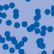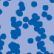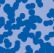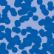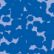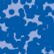## News

Prof. Dr. Karl-Theodor Sturm has been elected into the Academia Europaea. (28.06.2022)

Florian Schweiger erhielt den Hausdorff-Gedächtnispreis 2021 der Fachgruppe Mathematik für die beste Dissertation. Er fertigte die Dissertation unter der Betreuung von Prof. Stefan Müller an. Unter anderen wurde Vanessa Ryborz mit einem Preis der Bonner Mathematischen Gesellschaft für ihre von Prof. Sergio Conti betreute Bachelorarbeit ausgezeichnet. (18.01.2022)

Prof. Dr. Sergio Albeverio has been elected into the Academia Europaea and the Accademia Nazionale dei Lincei (more; 02.12.2021).

Der SFB 1060 Die Mathematik der emergenten Effekte hat eine dritte Förderperiode erhalten. (26.11.20)

Prof. Dr. Andreas Eberle erhält den diesjährigen Lehrpreis der Universität Bonn. (22.07.2020)

Herr Dr. Richard Höfer erhielt den Hausdorff-Gedächtnispreis 2019 der Fachgruppe Mathematik für die beste Disseration. Betreut wurde die Arbeit von Prof. J. Velázquez (29.01.2020).

Contact

Managing Director: Prof. Dr. Juan J. L. Velázquez